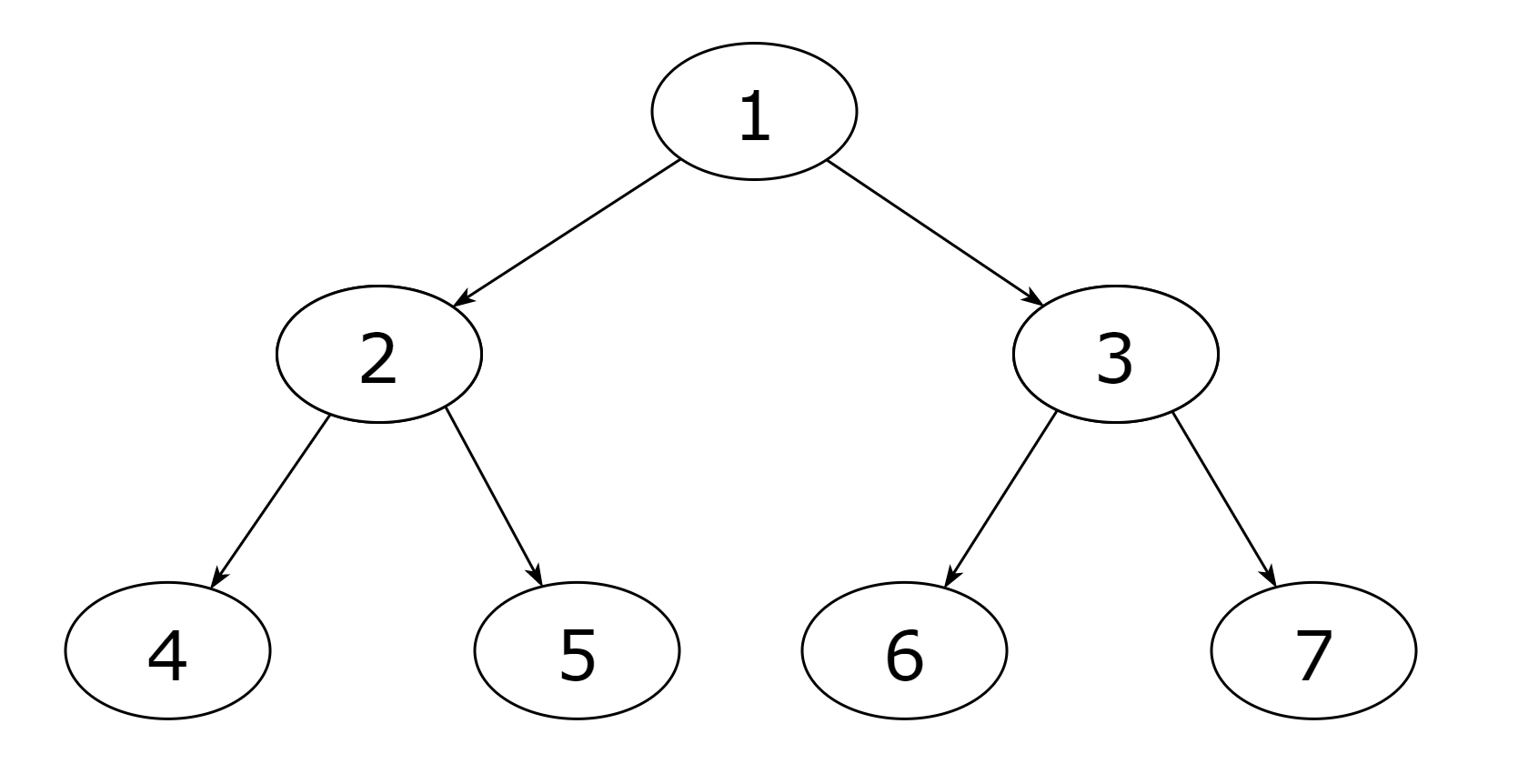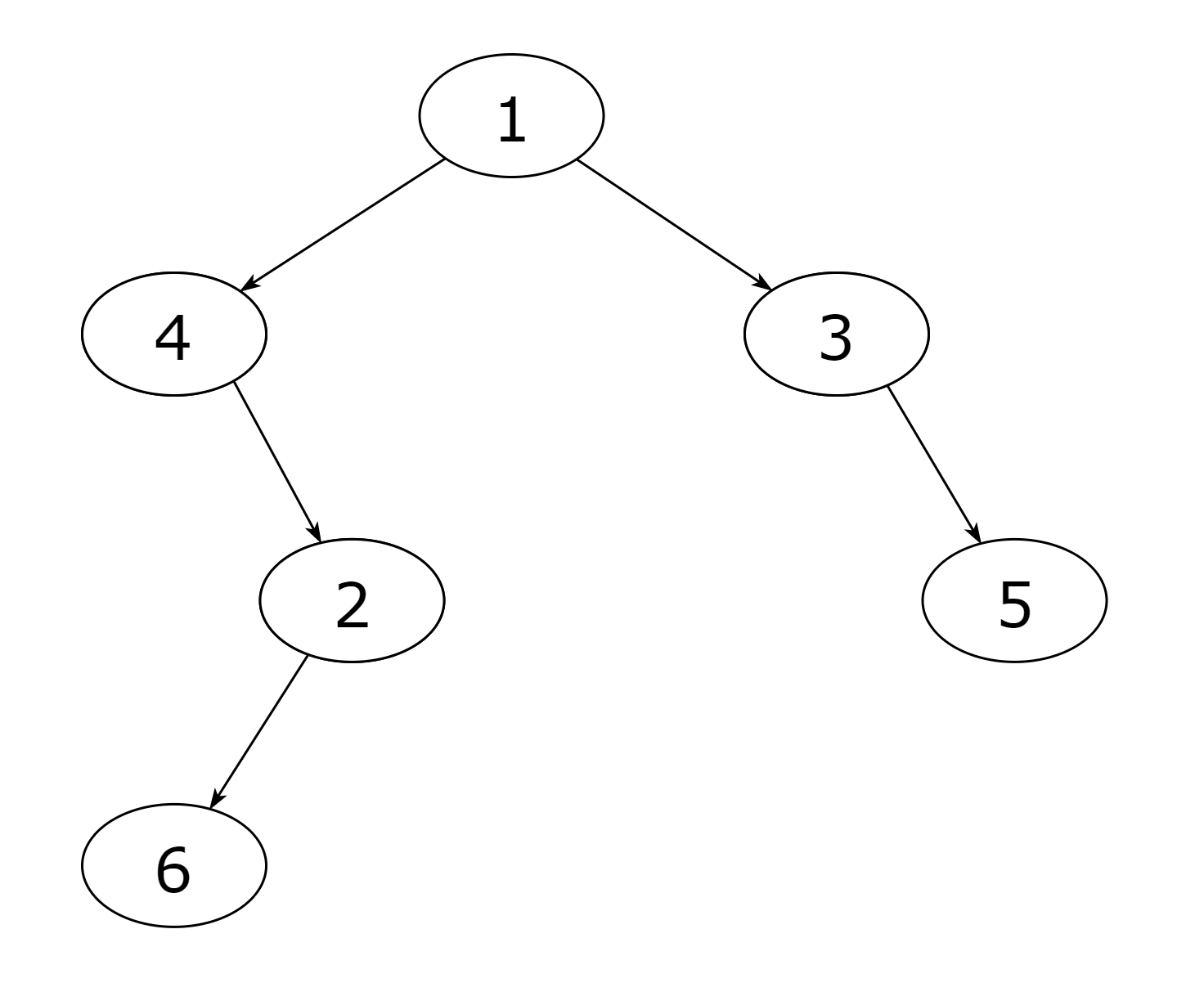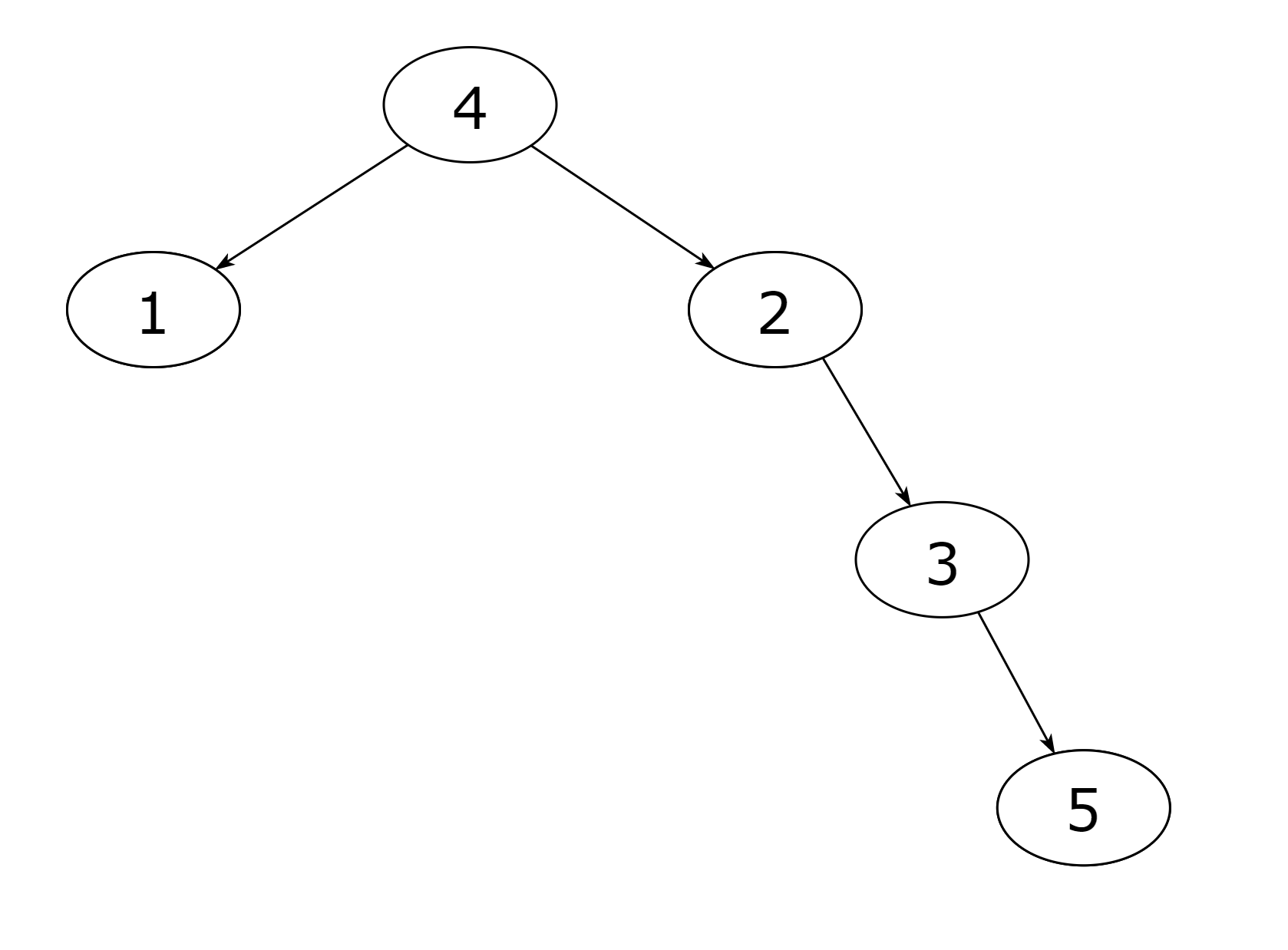Problem title
Difficulty
Avg time to solve

Three Ninja Candidate
Easy
10 mins
Minimum count of balls in a bag
Moderate
15 mins
LOOTCASE
Moderate
10 mins
Count of Matches in Tournament
Easy
20 mins
Flip Game II
Easy
15 mins
Baby Names
Moderate
15 mins
Number of squareful arrays
Hard
50 mins
Remove All Nodes with Value K
Easy
10 mins
Easy
10 mins
Insertion in AVL Tree
Moderate
20 mins5

# Create a binary tree from postorder and preorder traversal

Difficulty: MEDIUM
Avg. time to solve
15 min
Success Rate
85%

Problem Statement

#### You are given the ‘POSTORDER’ and ‘PREORDER’ traversals of a binary tree. The binary tree consists of ‘N’ nodes where each node represents a distinct positive integer named from ‘1’ to ‘N’. The task is to return the root node of any binary tree that matches the given ‘POSTORDER’ and ‘PREORDER’ traversals.

##### Example:
``````‘POSTORDER’ = [4, 5, 2, 6, 7, 3, 1]
‘PREORDER’ = [1, 2, 4, 5, 3, 6, 7]
A binary tree that matches the given ‘POSTORDER’ and ‘PREORDER’ traversal is:
````````````So, create this binary tree and return the root node ‘1’.
``````
##### Note:
``````1. You can return any binary tree that matches the given ‘POSTORDER’ and ‘PREORDER’ traversals.

2. You can always construct a valid binary tree from the ‘POSTORDER’ and ‘PREORDER’ traversals.
``````
##### Input format:
``````The first line of input contains an integer ‘T’ which denotes the number of test cases. Then, the ‘T’ test cases follow.

The first line of each test case contains an integer ‘N’ denoting the number of nodes in the binary tree.

The second line of each test case contains ‘N’ space-separated integers denoting the ‘POSTORDER’ traversal of the binary tree.

The third line of each test case contains ‘N’ space-separated integers denoting the ‘PREORDER’ traversal of the binary tree.
``````
##### Output format:
``````For every test case, return the root node of the newly created binary tree. The printed output will be the ‘POSTORDER’ and ‘PREORDER’ traversals of the returned tree, each printed on a new line.
``````
###### Note:
``````You do not need to print anything; it has already been taken care of. Just implement the function.
``````
##### Constraints:
``````1 <= T <= 10
1 <= N <= 10^3

Time limit: 1 second
``````

#### Sample input 1:

``````2
6
6 2 4 5 3 1
1 4 2 6 3 5
5
1 5 3 2 4
4 1 2 3 5
``````

#### Sample output 1:

``````6 2 4 5 3 1
1 4 2 6 3 5
1 5 3 2 4
4 1 2 3 5
``````

#### Explanation of sample input 1:

``````Test Case 1:

‘POSTORDER’ = [6, 2, 4, 5, 3, 1]
‘PREORDER’ = [1, 4, 2, 6, 3, 5]
A binary tree that matches the given ‘POSTORDER’ and ‘PREORDER’ traversal is:
````````````So, create this binary tree and return the root node ‘1’.

Test Case 2:

‘POSTORDER’ = [1, 5, 3, 2, 4]
‘PREORDER’ = [4, 1, 2, 3, 5]
A binary tree that matches the given ‘POSTORDER’ and ‘PREORDER’ traversal is:
````````````So, create this binary tree and return the root node ‘4’.
``````

#### Sample input 2:

``````2
4
3 4 1 2
2 1 3 4
5
5 4 3 2 1
1 2 3 4 5
``````

#### Sample output 2:

``````3 4 1 2
2 1 3 4
5 4 3 2 1
1 2 3 4 5
``````Console